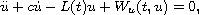Electron. J. Diff. Equ., Vol. 2015 (2015), No. 15, pp. 1-17.

### Homoclinic and quasi-homoclinic solutions for damped differential equations Chuan-Fang Zhang, Zhi-Qing Han

Abstract:
We study the existence and multiplicity of homoclinic solutions for the second-order damped differential equationwhere L(t) and W(t,u) are neither autonomous nor periodic in t. Under certain assumptions on L and W, we obtain infinitely many homoclinic solutions when the nonlinearity W(t,u) is sub-quadratic or super-quadratic by using critical point theorems. Some recent results in the literature are generalized, and the open problem proposed by Zhang and Yuan is solved. In addition, with the help of the Nehari manifold, we consider the case where W(t,u) is indefinite and prove the existence of at least one nontrivial quasi-homoclinic solution.

Submitted August 19, 2014. Published January 19, 2015.
Math Subject Classifications: 34C37, 35A15, 37J45.
Key Words: Homoclinic solution; Mountain pass theorem; damped differential equation; Nehari manifold.

Show me the PDF file (306 KB), TEX file, and other files for this article.Chuan-Fang Zhang School of Mathematical Sciences Dalian University of Technology 116024 Dalian, China email: kyzcf2006@163.com Zhi-Qing Han School of Mathematical Sciences Dalian University of Technology 116024 Dalian, China email: hanzhiq@dlut.edu.cn